College Physics for AP® Courses

# Test Prep for AP® Courses

College Physics for AP® CoursesTest Prep for AP® Courses

### 22.2Ferromagnets and Electromagnets

1.

A bar magnet is oriented so that the north pole of the bar magnet points north. A compass needle is placed to the north of the bar magnet. In which direction does the north pole of the compass needle point?

1. North
2. East
3. South
4. West
2.

Assume for simplicity that the Earth’s magnetic north pole is at the same location as its geographic north pole. If you are in an airplane flying due west along the equator, as you cross the prime meridian (0° longitude) facing west and look down at a compass you are carrying, you see that the compass needle is perpendicular to your direction of motion, and the north pole of the needle dipole points to your right. As you continue flying due west, describe how and why the orientation of the needle will (or will not) change.

3.

Describe how the magnetic domains in an unmagnetized iron rod will respond to the presence of a strong external magnetic field.

1. The domains will split into monopoles.
2. The domains will tend to align with the external field.
3. The domains will tend to orient themselves perpendicular to the external field.
4. The domains will tend to align so as to cancel out the external field.
4.

Describe what steps must be undertaken in order to convert an unmagnetized iron rod into a permanently magnetized state. As part of your answer, explain what a magnetic domain is and how it responds to the steps described.

5.

Iron is ferromagnetic and lead is diamagnetic, which means its magnetic domains respond in the opposite direction of ferromagnets but many orders of magnitude more weakly. The two blocks are placed in a magnetic field that points to the right. Which of the following best represents the orientations of the dipoles when the field is present?

 Iron Lead (a)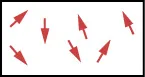Figure 22.71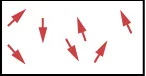Figure 22.72 (b)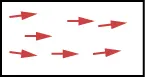Figure 22.73Figure 22.74 (c)Figure 22.75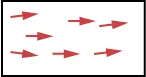Figure 22.76 (d)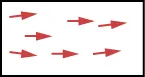Figure 22.77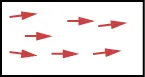Figure 22.78
Table 22.1
6.

A weather vane is some sort of directional arrow parallel to the ground that may rotate freely in a horizontal plane. A typical weather vane has a large cross-sectional area perpendicular to the direction the arrow is pointing, like a “One Way” street sign. The purpose of the weather vane is to indicate the direction of the wind. As wind blows past the weather vane, it tends to orient the arrow in the same direction as the wind. Consider a weather vane’s response to a strong wind. Explain how this is both similar to and different from a magnetic domain’s response to an external magnetic field. How does each affect its surroundings?

### 22.4Magnetic Field Strength: Force on a Moving Charge in a Magnetic Field

7.

A proton moves in the –x-direction and encounters a uniform magnetic field pointing in the +x-direction. In what direction is the resulting magnetic force on the proton?

1. The proton experiences no magnetic force.
2. +x-direction
3. y-direction
4. +y-direction
8.

A proton moves with a speed of 240 m/s in the +x-direction into a region of a 4.5-T uniform magnetic field directed 62° above the +x-direction in the x,y-plane. Calculate the magnitude of the magnetic force on the proton.

### 22.5Force on a Moving Charge in a Magnetic Field: Examples and Applications

9.

A wire oriented north-south carries current south. The wire is immersed in the Earth’s magnetic field, which is also oriented north-south (with a horizontal component pointing north). The Earth’s magnetic field also has a vertical component pointing down. What is the direction of the magnetic force felt by the wire?

1. West
2. East
3. Up
4. North

### 22.6The Hall Effect

10.

An airplane wingspan can be approximated as a conducting rod of length 35 m. As the airplane flies due north, it is flying at a rate of 82 m/s through the Earth’s magnetic field, which has a magnitude of 45 μT toward the north in a direction 57° below the horizontal plane. (a) Which end of the wingspan is positively charged, the east or west end? Explain. (b) What is the Hall emf along the wingspan?

### 22.9Magnetic Fields Produced by Currents: Ampere’s Law

11.

An experimentalist fires a beam of electrons, creating a visible path in the air that can be measured. The beam is fired along a direction parallel to a current-carrying wire, and the electrons travel in a circular path in response to the wire’s magnetic field. Assuming the mass and charge of the electrons is known, what quantities would you need to measure in order to deduce the current in the wire?

1. the radius of the circular path
2. the average distance between the electrons and the wire
3. the velocity of the electrons
4. two of the above
5. all of the above
12.

Electrons starting from rest are accelerated through a potential difference of 240 V and fired into a region of uniform 3.5-mT magnetic field generated by a large solenoid. The electrons are initially moving in the +x-direction upon entering the field, and the field is directed into the page. Determine (a) the radius of the circle in which the electrons will move in this uniform magnetic field and (b) the initial direction of the magnetic force the electrons feel upon entering the uniform field of the solenoid.

13.

In terms of the direction of force, we use the left-hand rule. Pointing your thumb in the +x-direction with the velocity and fingers of the left hand into the page reveals that the magnetic force points down toward the bottom of the page in the –y-direction.

A wire along the y-axis carries current in the +y-direction. In what direction is the magnetic field at a point on the +x-axis near the wire?

1. away from the wire
2. vertically upward
3. into the page
4. out of the page
14.

Imagine the xy coordinate plane is the plane of the page. A wire along the z-axis carries current in the +z-direction (out of the page, or $⊙ ⊙$ ). Draw a diagram of the magnetic field in the vicinity of this wire indicating the direction of the field. Also, describe how the strength of the magnetic field varies according to the distance from the z-axis.

### 22.10Magnetic Force between Two Parallel Conductors

15.

Two parallel wires carry equal currents in the same direction and are separated by a small distance. What is the direction of the magnetic force exerted by the two wires on each other?

1. No force since the wires are parallel.
2. No force since the currents are in the same direction.
3. The force is attractive.
4. The force is repulsive.
16.

A wire along the y-axis carries current in the +y-direction. An experimenter would like to arrange a second wire parallel to the first wire and crossing the x-axis at the coordinate so that the total magnetic field at the coordinate is zero. In what direction must the current flow in the second wire, assuming it is equal in magnitude to the current in the first wire? Explain.

Order a print copy

As an Amazon Associate we earn from qualifying purchases.